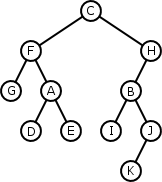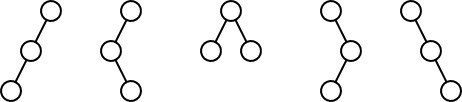# Binary Trees

## Why Care?

A binary tree is just a k-ary tree with k = 2. Because k is only 2,

• Binary trees are a bit simpler and easier to understand than trees with a large or unbounded number of children
• There are special traversals besides the usual breadth-first and depth-first traversals
• It is fun (or at least a valuable brain exercise) to generate the formula for the number of distinct binary tree shapes for a given number of nodes
• Binary tree nodes have an elegant linked representation with "left" and "right" subtrees
• Binary trees form the basis for efficient representations of sets, dictionaries, and priority queues

## Special Traversals

Like all oriented trees, breadth-first and depth-first traversals exist for binary trees, but there are others:• Preorder: C F G A D E H B I J K
• Inorder: G F D A E C I B K J H
• Postorder: G D E A F I K J B H C

## Binary Trees as Strings

Three simple rules:

• Write the empty tree as: `()`
• Write a non empty tree as: `(leftSubtree root rightSubtree)`
• An empty subtree can be, and usually is, omitted.

So for example:

• The tree with one element A is: `(() A ())`, or just `(A)`
• The tree with A as the root and B as the left child is: `((() B ()) A ())`, or just `((B) A)`
• The tree with A as the root and B as the right child is: `(() A (() B ()))`, or just `(A (B))`
• The tree in the previous section is: `(((G) F (D (A) E)) C (((I) B ((K) J)) H))`

## How Many Binary Trees Are There?

There are five distinct shapes of binary trees with three nodes:But how many are there for n nodes?

Let C(n) be the number of distinct binary trees with n nodes. This is equal to the number of trees that have a root, a left subtree with j nodes, and a right subtree of (n-1)-j nodes, for each j. That is,

```    C(n) = C(0)C(n-1) + C(1)C(n-2) + ... + C(n-1)C(0)
```

which isThe first few terms:

```    C(0) = 1
C(1) = C(0)C(0) = 1
C(2) = C(0)C(1) + C(1)C(0) = 2
C(3) = C(0)C(2) + C(1)C(1) + C(2)C(0) = 5
C(4) = C(0)C(3) + C(1)C(2) + C(2)C(1) + C(3)C(0) = 14
```

You can proveHere's the number of 8-node binary trees:

```           1   16!    16×15×14×13×12×11×10
C(8) = - × ---- = -------------------- = 13×11×10 = 1430
9   8!8!      8×7×6×5×4×3×2×1
```

## An Implementation of Binary Trees

As is common with trees (though quite unlike lists), node classes are visible to clients. Here is a simple binary tree node interface, again using the visitor pattern for traversals.

BinaryTreeNode.java
```package edu.lmu.cs.collections;

/**
* A simple interface for binary trees.  An empty binary tree is
* represented with the value null; a non-empty tree by its root
* node.
*/
public interface BinaryTreeNode<E> {

/**
* Returns the data stored in this node.
*/
E getData();

/**
* Modifies the data stored in this node.
*/
void setData(E data);

/**
* Returns the parent of this node, or null if this node is a root.
*/
BinaryTreeNode<E> getParent();

/**
* Returns the left child of this node, or null if it does
* not have one.
*/
BinaryTreeNode<E> getLeft();

/**
* Removes child from its current parent and inserts it as the
* left child of this node.  If this node already has a left
* child it is removed.
*
* @exception IllegalArgumentException if the child is
* an ancestor of this node, since that would make
* a cycle in the tree.
*/
void setLeft(BinaryTreeNode<E> child);

/**
* Returns the right child of this node, or null if it does
* not have one.
*/
BinaryTreeNode<E> getRight();

/**
* Removes child from its current parent and inserts it as the
* right child of this node.  If this node already has a right
* child it is removed.
* @exception IllegalArgumentException if the child is
* an ancestor of this node, since that would make
* a cycle in the tree.
*/
void setRight(BinaryTreeNode<E> child);

/**
* Removes this node, and all its descendants, from whatever
* tree it is in.  Does nothing if this node is a root.
*/
void removeFromParent();

/**
* Visits the nodes in this tree in preorder.
*/
void traversePreorder(Visitor visitor);

/**
* Visits the nodes in this tree in postorder.
*/
void traversePostorder(Visitor visitor);

/**
* Visits the nodes in this tree in inorder.
*/
void traverseInorder(Visitor visitor);

/**
* Simple visitor interface.
*/
public interface Visitor {
<E> void visit(BinaryTreeNode<E> node);
}
}```

We can implement this interface with links

```package edu.lmu.cs.collections;

/**
* An implementation of the BinaryTreeNode interface in which
* each node stores direct links to its left child, its right
* child, and its parent.
*
* <p>LinkedBinaryTreeNode objects are pretty mean: if one tries
* to mix them up with different kinds of binary tree nodes,
* and exception may be thrown.</p>
*/
public class LinkedBinaryTreeNode<E> implements BinaryTreeNode<E> {
protected E data;

/**
* Constructs a node as the root of its own one-element tree.
* This is the only public constructor.  The only trees that
* clients can make directly are simple one-element trees.
*/
public LinkedBinaryTreeNode(E data) {
this.data = data;
}

/**
* Returns the data stored in this node.
*/
public E getData() {
return data;
}

/**
* Modifies the data stored in this node.
*/
public void setData(E data) {
this.data = data;
}

/**
* Returns the parent of this node, or null if this node is a root.
*/
public BinaryTreeNode<E> getParent() {
return parent;
}

/**
* Returns the left child of this node, or null if it does
* not have one.
*/
public BinaryTreeNode<E> getLeft() {
return left;
}

/**
* Removes child from its current parent and inserts it as the
* left child of this node.  If this node already has a left
* child it is removed.
* @exception IllegalArgumentException if the child is
* an ancestor of this node, since that would make
* a cycle in the tree.
*/
public void setLeft(BinaryTreeNode<E> child) {
// Ensure the child is not an ancestor.
for (LinkedBinaryTreeNode<E> n = this; n != null; n = n.parent) {
if (n == child) {
throw new IllegalArgumentException();
}
}

// Ensure that the child is an instance of LinkedBinaryTreeNode.

// Break old links, then reconnect properly.
if (this.left != null) {
left.parent = null;
}
if (childNode != null) {
childNode.removeFromParent();
childNode.parent = this;
}
this.left = childNode;
}

/**
* Returns the right child of this node, or null if it does
* not have one.
*/
public BinaryTreeNode<E> getRight() {
return right;
}

/**
* Removes child from its current parent and inserts it as the
* right child of this node.  If this node already has a right
* child it is removed.
* @exception IllegalArgumentException if the child is
* an ancestor of this node, since that would make
* a cycle in the tree.
*/
public void setRight(BinaryTreeNode<E> child) {
// Ensure the child is not an ancestor.
for (LinkedBinaryTreeNode<E> n = this; n != null; n = n.parent) {
if (n == child) {
throw new IllegalArgumentException();
}
}

// Ensure that the child is an instance of LinkedBinaryTreeNode.

// Break old links, then reconnect properly.
if (right != null) {
right.parent = null;
}
if (childNode != null) {
childNode.removeFromParent();
childNode.parent = this;
}
this.right = childNode;
}

/**
* Removes this node, and all its descendants, from whatever
* tree it is in.  Does nothing if this node is a root.
*/
public void removeFromParent() {
if (parent != null) {
if (parent.left == this) {
parent.left = null;
} else if (parent.right == this) {
parent.right = null;
}
this.parent = null;
}
}

/**
* Visits the nodes in this tree in preorder.
*/
public void traversePreorder(BinaryTreeNode.Visitor visitor) {
visitor.visit(this);
if (left != null) left.traversePreorder(visitor);
if (right != null) right.traversePreorder(visitor);
}

/**
* Visits the nodes in this tree in postorder.
*/
public void traversePostorder(Visitor visitor) {
if (left != null) left.traversePostorder(visitor);
if (right != null) right.traversePostorder(visitor);
visitor.visit(this);
}

/**
* Visits the nodes in this tree in inorder.
*/
public void traverseInorder(Visitor visitor) {
if (left != null) left.traverseInorder(visitor);
visitor.visit(this);
if (right != null) right.traverseInorder(visitor);
}
}```

For fun, here is a panel class that can draw a binary tree.

BinaryTreePanel.java
```package edu.lmu.cs.collections;

import java.awt.Color;
import java.awt.FontMetrics;
import java.awt.Graphics;
import java.awt.Point;
import java.awt.Rectangle;
import java.lang.reflect.Field;
import java.util.HashMap;
import java.util.Map;

import javax.swing.JPanel;

/**
* A panel that maintains a picture of a binary tree.
*/
public class BinaryTreePanel extends JPanel {
private BinaryTreeNode<?> tree;
private int gridwidth;
private int gridheight;

/**
* Stores the pixel values for each node in the tree.
*/
private Map<BinaryTreeNode<?>, Point> coordinates =
new HashMap<BinaryTreeNode<?>, Point>();

/**
* Constructs a panel, saving the tree and drawing parameters.
*/
public BinaryTreePanel(BinaryTreeNode<?> tree, int gridwidth, int gridheight) {
this.tree = tree;
this.gridwidth = gridwidth;
this.gridheight = gridheight;
}

/**
* Changes the tree rendered by this panel.
*/
public void setTree(BinaryTreeNode<?> root) {
tree = root;
repaint();
}

/**
* Draws the tree in the panel.  First it computes the coordinates
* of all the nodes with an inorder traversal, then draws them
* with a postorder traversal.
*/
public void paintComponent(final Graphics g) {
super.paintComponent(g);

if (tree == null) {
return;
}

tree.traverseInorder(new BinaryTreeNode.Visitor() {
private int x = gridwidth;
public void visit(BinaryTreeNode node) {
coordinates.put(node, new Point(x, gridheight * (depth(node)+1)));
x += gridwidth;
}
});

tree.traversePostorder(new BinaryTreeNode.Visitor() {
public void visit(BinaryTreeNode node) {
String data = node.getData().toString();
Point center = (Point)coordinates.get(node);
if (node.getParent() != null) {
Point parentPoint = (Point)coordinates.get(node.getParent());
g.setColor(Color.black);
g.drawLine(center.x, center.y, parentPoint.x, parentPoint.y);
}
FontMetrics fm = g.getFontMetrics();
Rectangle r = fm.getStringBounds(data, g).getBounds();
r.setLocation(center.x - r.width/2, center.y - r.height/2);
Color color = getNodeColor(node);
Color textColor =
(color.getRed() + color.getBlue() + color.getGreen() < 382)
? Color.white
: Color.black;
g.setColor(color);
g.fillRect(r.x - 2 , r.y - 2, r.width + 4, r.height + 4);
g.setColor(textColor);
g.drawString(data, r.x, r.y + r.height);
}
});
}

/**
* Returns a color for the node.  If the node is of a class with a
* field called "color", and that field currently contains a
* non-null value, then that value is returned.  Otherwise
* a default color of yellow is returned.
*/
Color getNodeColor(BinaryTreeNode<?> node) {
try {
Field field = node.getClass().getDeclaredField("color");
return (Color)field.get(node);
} catch (Exception e) {
return Color.yellow;
}
}

private int depth(BinaryTreeNode<?> node) {
return (node.getParent() == null) ? 0 : 1 + depth(node.getParent());
}
}```